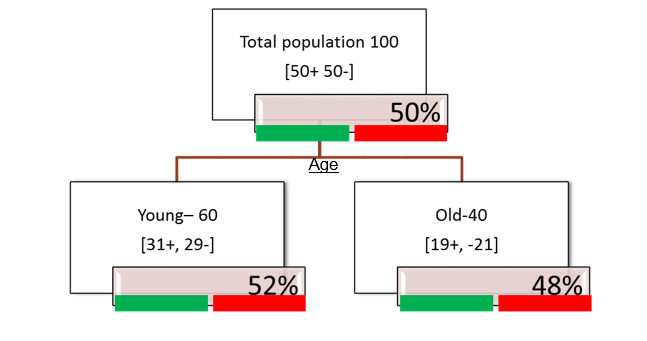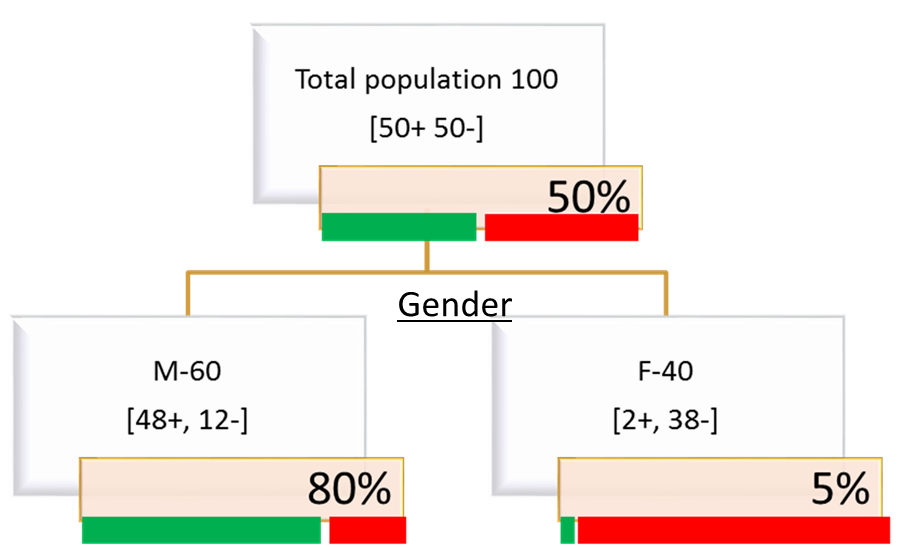• No products in the cart.

# 204.3.4 How to Calculate Entropy for Decision Tree Split?

##### Some math behind Decision Trees.
Link to the previous post : https://statinfer.com/204-3-3-how-decision-tree-splits-works/

## Entropy Calculation – Example

• Entropy at root
• Total population at root 100 [50+,50-]
• Entropy(S) = p+log2p+plog2p
• 0.5log2(0.5)0.5log2(0.5)
• -(0.5)(-1) – (0.5)(-1)
• 1
• 100% Impurity at root

Entropy(S)=−(p+)(log2(p+))−(p−)(log2(p−))### Entropy Calculation

• Gender Splits the population into two segments
• Segment-1 : Age=”Young”
• Segment-2: Age=”Old”
• Entropy at segment-1
• Age=”Young” segment has 60 records [31+,29-]
Entropy(S)=−(p+)(log2(p+))−(p−)(log2(p−))
• 31/60log231/6029/60log229/60
• (-31/60)log(31/60,2)-(29/60)log(29/60,2)
• 0.9991984 (99% Impurity in this segment)• Entropy at segment-2
• Age=”Old” segment has 40 records [19+,21-]
Entropy(S)=−(p+)(log2(p+))−(p−)(log2(p−))
• 19/40log219/4021/40log221/40
• (-19/40)log(19/40,2)-(21/40)log(21/40,2)
• 0.9981959(99% Impurity in this segment too)

### Practice : Entropy Calculation – Example

• Calculate entropy at the root for the given population
• Calculate the entropy for the two distinct gender segments### Code- Entropy Calculation

• Entropy at root 100%
• Male Segment : (-48/60)log(48/60,2)-(12/60)log(12/60,2)
• 0.7219281
• FemaleSegment : (-2/40)log(2/40,2)-(38/40)log(38/40,2)
• 0.286397The next post is about information gain in decision tree split.
24th January 2018
0 responses on "204.3.4 How to Calculate Entropy for Decision Tree Split?"